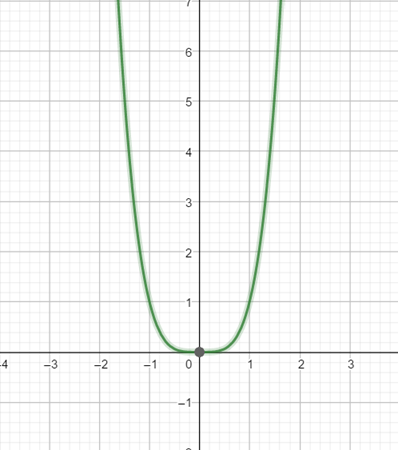# To state : whether the function f ( x ) = x 4 is even, odd or neither.### Precalculus: Mathematics for Calcu...

6th Edition
Stewart + 5 others
Publisher: Cengage Learning
ISBN: 9780840068071### Precalculus: Mathematics for Calcu...

6th Edition
Stewart + 5 others
Publisher: Cengage Learning
ISBN: 9780840068071

#### Solutions

Chapter 2.5, Problem 75E
To determine

## To state : whether the function f(x)=x4 is even, odd or neither.

Expert Solution

The function f(x)=x4 is even.

### Explanation of Solution

Given information :

The function is f(x)=x4

The function f is even if f(x)=f(x) for all x in the domain of f .

The function f is odd if f(x)=f(x) for all x in the domain of f .

f(x)=x4f(x)=(x)4f(x)=x4f(x)=f(x)

Therefore, f(x)=x4 is an even function.

Hence, the graph of f(x)=x4 is symmetric about the y -axis,### Have a homework question?

Subscribe to bartleby learn! Ask subject matter experts 30 homework questions each month. Plus, you’ll have access to millions of step-by-step textbook answers!